### 光线追踪的历史### 光线特性

• 更精确的反射、折射和透射。
• 更准确的阴影。包括自阴影、软阴影、区域阴影、多光源阴影等。
• 更精准的全局光照。
• 更真实的环境光遮蔽（AO）

## 光线追踪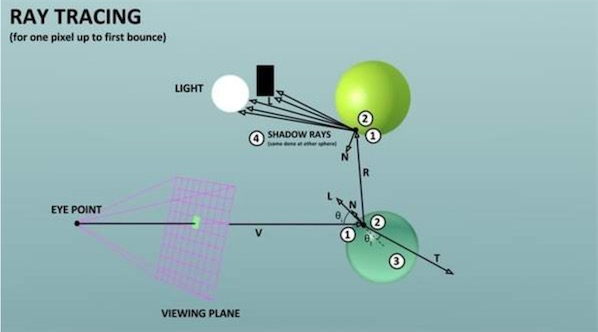#### 基本步骤


public class RayTracingMaster : MonoBehaviour
{
public ComputeShader RayTracingShader;
private RenderTexture _target;

private void OnRenderImage(RenderTexture source, RenderTexture destination) {
Render(destination);
}

private void Render(RenderTexture destination) {
InitRenderTexture();
RayTracingShader.SetTexture(0, "Result", _target);
int threadGroupsX = Mathf.CeilToInt(Screen.width / 8.0f);
int threadGroupsY = Mathf.CeilToInt(Screen.height / 8.0f);
RayTracingShader.Dispatch(0, threadGroupsX, threadGroupsY, 1);
Graphics.Blit(_target, destination);
}

private void InitRenderTexture() {
if (_target == null || _target.width != Screen.width
|| _target.height != Screen.height) {
if (_target != null)
_target.Release();

_target = new RenderTexture(Screen.width, Screen.height, 0,
RenderTextureFormat.ARGBFloat, RenderTextureReadWrite.Linear);
_target.enableRandomWrite = true;
_target.Create();
}
}
}


compute shader 需要传递一些camera的参数给compute shader, 主要是用来空间变换

• cameraToWorldMatrix 观察坐标系切换到世界坐标系
• projectionMatrix 观察坐标系转到投影坐标系 inverse是反过来，即投影坐标系变换到观察坐标系
traceShader.SetMatrix("_CameraToWorld", _camera.cameraToWorldMatrix);
traceShader.SetMatrix("_CameraInverseProjection", _camera.projectionMatrix.inverse);


#pragma kernel CSMain

RWTexture2D<float4> Result;
float4x4 _CameraToWorld;
float4x4 _CameraInverseProjection;

struct Ray {
float3 origin;
float3 direction;
};

Ray CreateRay(float3 origin, float3 direction) {
Ray ray;
ray.origin = origin;
ray.direction = direction;
return ray;
}

Ray CreateCameraRay(float2 uv) {
// 观察空间到世界空间变换
float3 origin = mul(_CameraToWorld, float4(0.0f, 0.0f, 0.0f, 1.0f)).xyz;
// 投影空间变换到观察空间
float3 direction = mul(_CameraInverseProjection, float4(uv, 0.0f, 1.0f)).xyz;
// 变换到时间空间 w=0只变换方向
direction = mul(_CameraToWorld, float4(direction, 0.0f)).xyz;
direction = normalize(direction);
return CreateRay(origin, direction);
}

[numthreads(8,8,1)]
void CSMain (uint3 id : SV_DispatchThreadID) {
// 获取RT分辨率
uint width, height;
Result.GetDimensions(width, height);
// [0,1] 变换到 [-1,1]
float2 uv = float2((id.xy + float2(0.5f, 0.5f))/float2(width, height)*2.0f-1.0f);
Ray ray = CreateCameraRay(uv);
Result[id.xy] = float4(ray.direction * 0.5f + 0.5f, 1.0f);
}


//因为我们天空盒导入的是2D的，所以要把方向转为球坐标系来采样
float theta = acos(ray.direction.y) / -PI;
float phi = atan2(ray.direction.x, -ray.direction.z) / -PI * 0.5f;
return _SkyboxTexture.SampleLevel(sampler_SkyboxTexture, float2(phi, theta), 0).rgb;


#### 光线追踪流程

//光线进行7次反弹
float3 result=float3(0.0f,0.0f,0.0f);
for(int i=0;i<8;i++) {
RayHit hit=Trace(ray);
result+=ray.energy*Shade(ray,hit);
//光线能量耗尽直接退出循环
if(!any(ray.energy)) break;
}


//y=0的平面的射线相交检测
void IntersectGroundPlane(Ray ray,inout RayHit bestHit) {
float t=-ray.origin.y/ray.direction.y;
if(t>0&&t<bestHit.distance) {
bestHit.distance=t;
bestHit.position=ray.origin+t*ray.direction;
bestHit.normal=float3(0,1,0);
bestHit.albedo=float3(1.0f,1.0f,1.0f);
bestHit.specular=float3(0.2f,0.2f,0.2f);
}
}

//球的相交检测
void IntersectSphere(Ray ray, inout RayHit bestHit, Sphere sphere) {
float3 d = ray.origin - sphere.position;
float p1 = -dot(ray.direction, d);
float p2sqr = p1 * p1 - dot(d, d) + sphere.radius * sphere.radius;
if (p2sqr < 0) //不想交的情况
return;
float p2 = sqrt(p2sqr);
float t = p1 - p2 > 0 ? p1 - p2 : p1 + p2;
if (t > 0 && t < bestHit.distance) {
bestHit.distance = t;
bestHit.position = ray.origin + t * ray.direction;
bestHit.normal = normalize(bestHit.position - sphere.position);
bestHit.albedo=sphere.albedo;
bestHit.specular=sphere.specular;
}
}


float3 Shade(inout Ray ray,RayHit hit) {
if(hit.distance<1.#INF) {
ray.origin=hit.position+hit.normal*0.001f;
ray.direction=reflect(ray.direction,hit.normal);
ray.energy*=hit.specular;
return float3(0.0f,0.0f,0.0f)
}else{
ray.energy=0;
//没有碰撞的物体就采样天空盒
//因为我们天空盒导入的是2D的，所以要把方向转为极坐标来采样
float theta = acos(ray.direction.y) / -PI;
float phi = atan2(ray.direction.x, -ray.direction.z) / -PI * 0.5f;
return _SkyboxTexture.SampleLevel(sampler_SkyboxTexture, float2(phi, theta), 0).rgb;
}
}


return saturate(dot(hit.normal,_DirectionLight.xyz)*-1)*_DirectionLight.w*hit.albedo;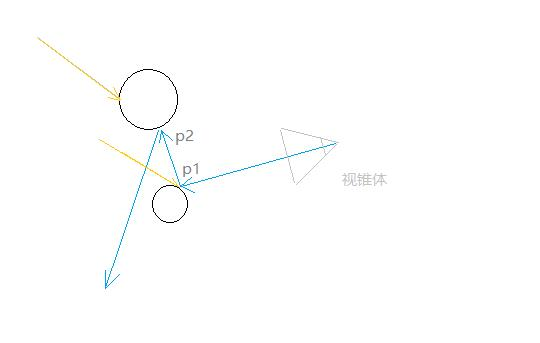//阴影检测，向直射光的反方向进行射线检测，如果撞到了物体，就说明他在直射光的阴影之下，返回0
Ray shadowRay = CreateRay(hit.position + hit.normal * 0.001f, -1 * _DirectionLight.xyz);
RayHit shadowHit = Trace(shadowRay);
if (shadowHit.distance != 1.#INF)


//映射至uv的01范围内
float2 uv=float2((id.xy+_PixelOffset.xy)/float2(width,height)*2.0f-1.0f);


Cull Off ZWrite Off ZTest Always
Blend SrcAlpha OneMinusSrcAlpha
Pass
{
CGPROGRAM
#pragma vertex vert
#pragma fragment frag
#pragma multi_compile_fog

#include "UnityCG.cginc"

struct appdata {
float4 vertex : POSITION;
float2 uv : TEXCOORD0;
};

struct v2f {
float2 uv : TEXCOORD0;
UNITY_FOG_COORDS(1)
float4 vertex : SV_POSITION;
};

sampler2D _MainTex;
float4 _MainTex_ST;
float _Sample;

v2f vert (appdata v) {
v2f o;
o.vertex = UnityObjectToClipPos(v.vertex);
o.uv = TRANSFORM_TEX(v.uv, _MainTex);
UNITY_TRANSFER_FOG(o,o.vertex);
return o;
}

float4 frag (v2f i) : SV_Target {
return float4(tex2D(_MainTex, i.uv).rgb, 1.0f / (_Sample + 1.0f));
}
ENDCG
}


## 路径追踪

#### 渲染方程

$L_o(x,\vec{w_o})$：碰撞点X在w方向的出射光

$L_e(x,\vec{w_o} )$: 出射光的自发光贡献

$\int _\Omega …$: Ω=法线方向的半球面，这个积分的意思就是综合这个半球面所有可能方向的入射光对这个指定的出射光线的贡献。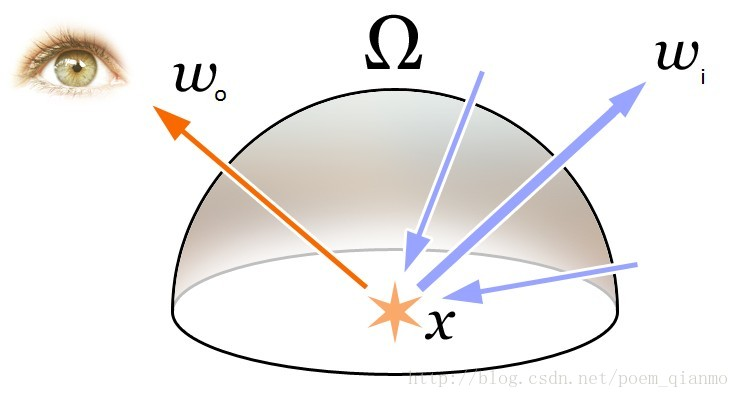$f_r(x,\vec{w_i},\vec{w_o})$：双向反射分布函数BRDF

$\vec{w_i }\cdot \vec{n}$: 入射光和表面法线的夹角cos值，夹角越大，值越低，出射光强度越弱，因为夹角越大大，一束光线越容易分散

$L(x,\vec{w_i})$: 入射光，这个值就是前一个碰撞点用渲染方程得到的出射光，如此递归直到结束。

#### 蒙特卡洛积分

GPU里没有类似random()函数之类的算法， 需要自己去实现， 下面是网上的产生随机值算法。

float2 _Pixel;
float _Seed;
float rand()
{
float result = frac(sin(_Seed / 100.0f * dot(_Pixel, float2(12.9898f, 78.233f))) * 43758.5453f);
_Seed += 1.0f;
return result;
}


_Pixel直接在CSMain里初始化： _Pixel=id.xy, 所以每个像素都使用不同的随机值。 _Seed是c#侧传过来的初始值，这里当做随机种子使用。

Lambert BRDF: $f_r(x, \, \vec \omega_{i}, \, \vec \omega_{o}) = \frac{k_d}{\pi}$, 其中$k_d$代表着材质的albeo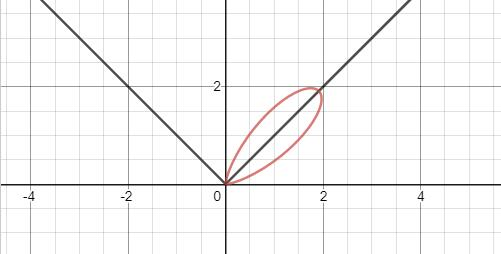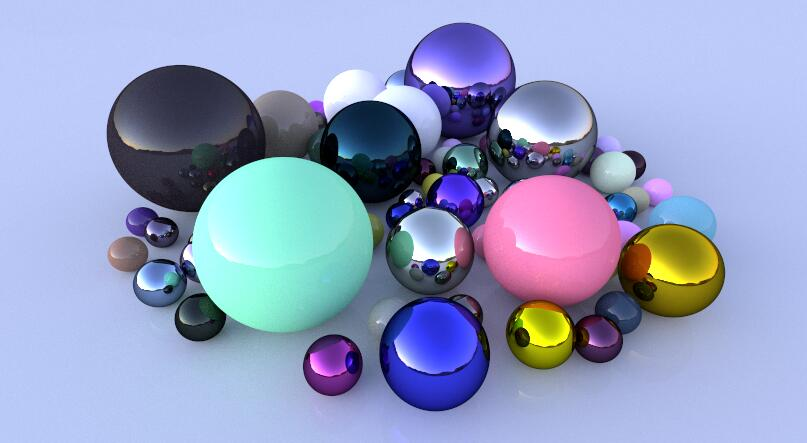### Mesh 碰撞检测

private void RebuildMeshObjectBuffers() {
if (!_meshObjectsNeedRebuilding) return;

_meshObjectsNeedRebuilding = false;
_currentSample = 0;
_meshObjects.Clear();
_vertices.Clear();
_indices.Clear();

// Loop over all objects and gather their data
foreach (RayTracingObject obj in _rayTracingObjects) {
Mesh mesh = obj.GetComponent<MeshFilter>().sharedMesh;

int firstVertex = _vertices.Count;
_vertices.AddRange(mesh.vertices);

int firstIndex = _indices.Count;
var indices = mesh.GetIndices(0);
_indices.AddRange(indices.Select(index => index + firstVertex));

_meshObjects.Add(new MeshObject() {
localToWorldMatrix = obj.transform.localToWorldMatrix,
indices_offset = firstIndex,
indices_count = indices.Length
});
}

CreateComputeBuffer(ref _meshObjectBuffer, _meshObjects, 72);
CreateComputeBuffer(ref _vertexBuffer, _vertices, 12);
CreateComputeBuffer(ref _indexBuffer, _indices, 4);
}


shader 中判断三角形是否与射线相交的算法， 在文章后面的部分会介绍推导， Mesh是由大量的三角形构成，我们遍历mesh里的每个三角形， 这里用来判断射线和Mesh相交。

void IntersectMeshObject(Ray ray, inout RayHit bestHit, MeshObject meshObject)
{
uint offset = meshObject.indices_offset;
uint count = offset + meshObject.indices_count;
for (uint i = offset; i < count; i += 3) {
float4x4 local2world = meshObject.localToWorldMatrix;
float3 v0 = (mul(local2world, float4(_Vertices[_Indices[i]], 1))).xyz;
float3 v1 = (mul(local2world, float4(_Vertices[_Indices[i + 1]], 1))).xyz;
float3 v2 = (mul(local2world, float4(_Vertices[_Indices[i + 2]], 1))).xyz;

float t, u, v;
if (IntersectTriangle_MT97(ray, v0, v1, v2, t, u, v)) {
if (t > 0 && t < bestHit.distance) {
bestHit.distance = t;
bestHit.position = ray.origin + t * ray.direction;
bestHit.normal = normalize(cross(v1 - v0, v2 - v0));
bestHit.albedo = 0.0f;
bestHit.specular = 0.65f;
bestHit.smoothness = 0.99f;
bestHit.emission = 0.0f;
}
}
}
}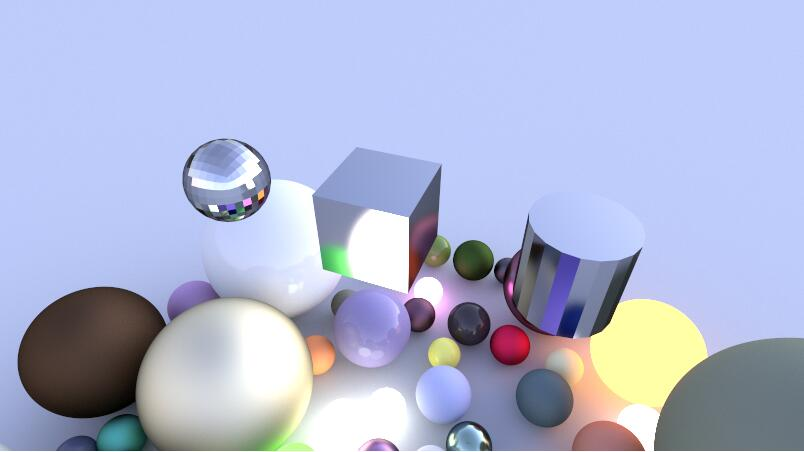## 射线相交检测算法

#### 1. 射线与平面相交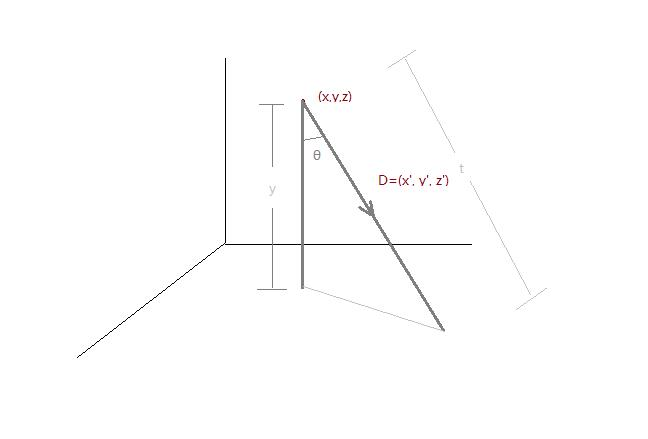bool IntersectPlane(Ray ray) {
t = -ray.origin.y / ray.direction.y;
return true;
}


#### 2. 射线与球相交

<0无解 =0 一解 >0 两解 $t=P_1\pm P_2$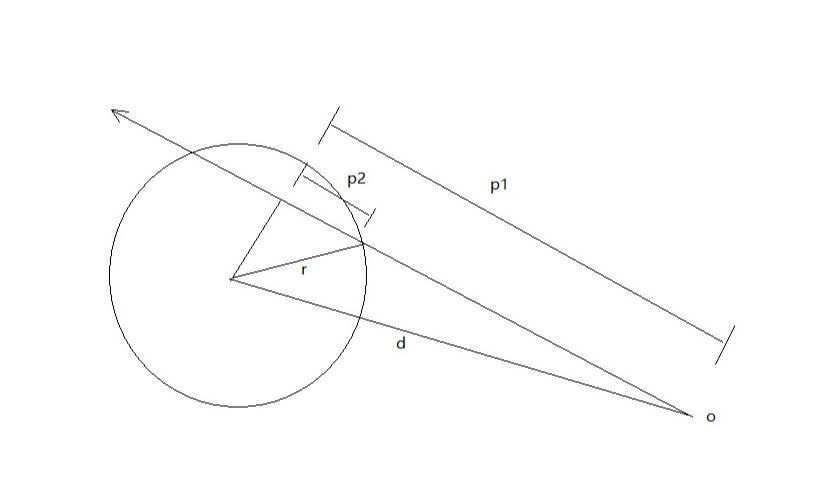bool IntersectSphere(Ray ray, float3 position,float radius,inout float t) {
float3 d = ray.origin - position;
float p1 = -dot(ray.direction, d);
float p2sqr = p1 * p1 - dot(d, d) +radius*radius;
if (p2sqr < 0)//不存在解也就是不相交
return false;
float p2 = sqrt(p2sqr);
t = p1 - p2 > 0 ? p1 - p2 : p1 + p2;//优先选近的那个点，除非近的点在后头
return true;
}


#### 3. 射线与三角形相交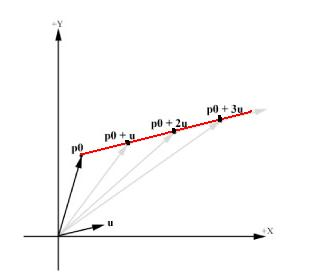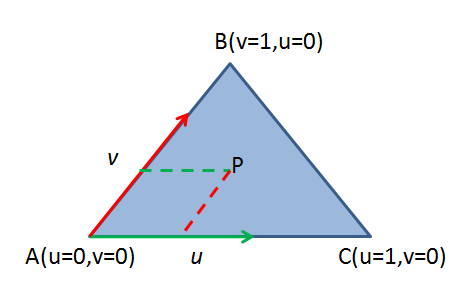//三角形的相交性检测 双面 uvw 重心坐标 w=1-u-v  t=距离
static const float EPSILON = 1e-8;
bool IntersectTriangle_01(Ray ray, float3 vert0, float3 vert1, float3 vert2,
inout float t, inout float u, inout float v,inout float toward) {
//E1 E2
float3 edge1 = vert1 - vert0;
float3 edge2 = vert2 - vert0;
//P=DxE2
float3 pvec = cross(ray.direction, edge2);
float det = dot(edge1, pvec);
//背面det<0 正面>0 在三角形内=0
if (det < EPSILON&&det >-EPSILON) return false;
toward=sign(det);
// 1/(DxE2)·E1
float inv_det = 1.0f / det;
//T
float3 tvec = ray.origin - vert0;
//u=invdet *(P·T)
u = dot(tvec, pvec) * inv_det;
//如果三角形内的点，重心坐标一定在[0,1]
if (u < 0.0 || u > 1.0f) return false;
//Q=TxE1
float3 qvec = cross(tvec, edge1);
//v=invdet*(Q·D)
v = dot(ray.direction, qvec) * inv_det;
if (v < 0.0 || u + v > 1.0f) return false;
//t=Q·E2
t = dot(edge2, qvec) * inv_det;
return true;
}


#### 4. 射线与立方盒相交

① 寻找可能正面相交的平面（最多三个）

$D_x>0$:光线与平面x=0相交

$D_x<0$：光线与平面$x=r_x$相交

$D_x=0$ ：两个面都不相交

② 计算与平面的焦点，得到最近的相交点（在矩形内部）

#define ISPOINT(name,c1,c2) name.c1>=0&&name.c1<=cubeSize.c1&& name.c2>=0&&name.c2<=cubeSize.c2
//立方体 对象空间
bool IntersectCube(Ray ray,float3 cubeSize,inout float t,inout float3 normal){
//1.用矩阵将射线转为立方体的对象空间，略 后面的法线也应该转回世界空间
float3 rayOri=ray.origin;
float3 rayDir=ray.direction;
float3 pos;
//x轴两个面
if(rayDir.x<0){
t=(cubeSize.x-rayOri.x)/rayDir.x;
pos=rayOri+t*rayDir;
if(ISPOINT(pos,y,z)){
normal=float3(1,0,0);
return true;
}
}
else if(rayDir.x>0){
t=-rayOri.x/rayDir.x;
pos=rayOri+t*rayDir;
if(ISPOINT(pos,y,z)){
normal=float3(-1,0,0);
return true;
}
}
//y轴两个面
if(rayDir.y<0){
t=(cubeSize.y-rayOri.y)/rayDir.y;
pos=rayOri+t*rayDir;
if(ISPOINT(pos,x,z)){
normal=float3(0,1,0);
return true;
}
}
else if(rayDir.y>0){
t=-rayOri.y/rayDir.y;
pos=rayOri+t*rayDir;
if(ISPOINT(pos,x,z)){
normal=float3(0,-1,0);
return true;
}
}
//z轴两个面
if(rayDir.z<0){
t=(cubeSize.z-rayOri.z)/rayDir.z;
pos=rayOri+t*rayDir;
if(ISPOINT(pos,x,y)){
normal=float3(0,0,1);
return true;
}
}
else if(rayDir.z>0){
t=-rayOri.z/rayDir.z;
pos=rayOri+t*rayDir;
if(ISPOINT(pos,x,y)){
normal=float3(0,0,-1);
return true;
}
}
return false;
}